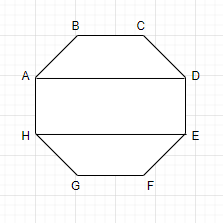QUESTION

# Draw a rough sketch of a regular octagon. (Use squared paper if you wish). Draw a rectangle by joining exactly four of the vertices of the octagon.

Hint – Here we will proceed by constructing an octagon such that all sides of the octagon are equal. Then, we will join four vertices which is the property of a regular octagon. Hence we will get the desired result.$\Rightarrow$ This implies that all the sides of the octagon are equal.